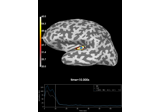# mne.minimum_norm.compute_source_psd_epochs¶

mne.minimum_norm.compute_source_psd_epochs(epochs, inverse_operator, lambda2=0.1111111111111111, method='dSPM', fmin=0.0, fmax=200.0, pick_ori=None, label=None, nave=1, pca=True, inv_split=None, bandwidth=4.0, adaptive=False, low_bias=True, return_generator=False, n_jobs=1, prepared=False, method_params=None, return_sensor=False, use_cps=True, verbose=None)[source]

Compute source power spectral density (PSD) from Epochs.

This uses the multi-taper method to compute the PSD for each epoch.

Parameters
epochsinstance of `Epochs`

The raw data.

inverse_operatorinstance of `InverseOperator`

The inverse operator.

lambda2`float`

The regularization parameter.

method“MNE” | “dSPM” | “sLORETA” | “eLORETA”

Use minimum norm, dSPM (default), sLORETA, or eLORETA.

fmin`float`

The lower frequency of interest.

fmax`float`

The upper frequency of interest.

pick_ori`None` | “normal”

If “normal”, rather than pooling the orientations by taking the norm, only the radial component is kept. This is only implemented when working with loose orientations.

label`Label`

Restricts the source estimates to a given label.

nave`int`

The number of averages used to scale the noise covariance matrix.

pcabool

If True, the true dimension of data is estimated before running the time-frequency transforms. It reduces the computation times e.g. with a dataset that was maxfiltered (true dim is 64).

inv_split

Split inverse operator into inv_split parts in order to save memory.

bandwidth

The bandwidth of the multi taper windowing function in Hz. Can also be a string (e.g., ‘hann’) to use a single window.

Use adaptive weights to combine the tapered spectra into PSD (slow, use n_jobs >> 1 to speed up computation).

low_biasbool

Only use tapers with more than 90% spectral concentration within bandwidth.

return_generatorbool

Return a generator object instead of a list. This allows iterating over the stcs without having to keep them all in memory.

n_jobs`int`

The number of jobs to run in parallel (default 1). Requires the joblib package. It is only used if adaptive=True.

preparedbool

If True, do not call `prepare_inverse_operator()`.

method_params

Additional options for eLORETA. See Notes of `apply_inverse()`.

New in version 0.16.

return_sensorbool

If True, also return the sensor PSD for each epoch as an EvokedArray.

New in version 0.17.

use_cpsbool

Whether to use cortical patch statistics to define normal orientations for surfaces (default True).

Only used when the inverse is free orientation (`loose=1.`), not in surface orientation, and `pick_ori='normal'`.

New in version 0.20.

verbose

If not None, override default verbose level (see `mne.verbose()` and Logging documentation for more). If used, it should be passed as a keyword-argument only.

Returns
out`list` (or generator object)

A list (or generator) for the source space PSD (and optionally the sensor PSD) for each epoch.

## Examples using `mne.minimum_norm.compute_source_psd_epochs`¶